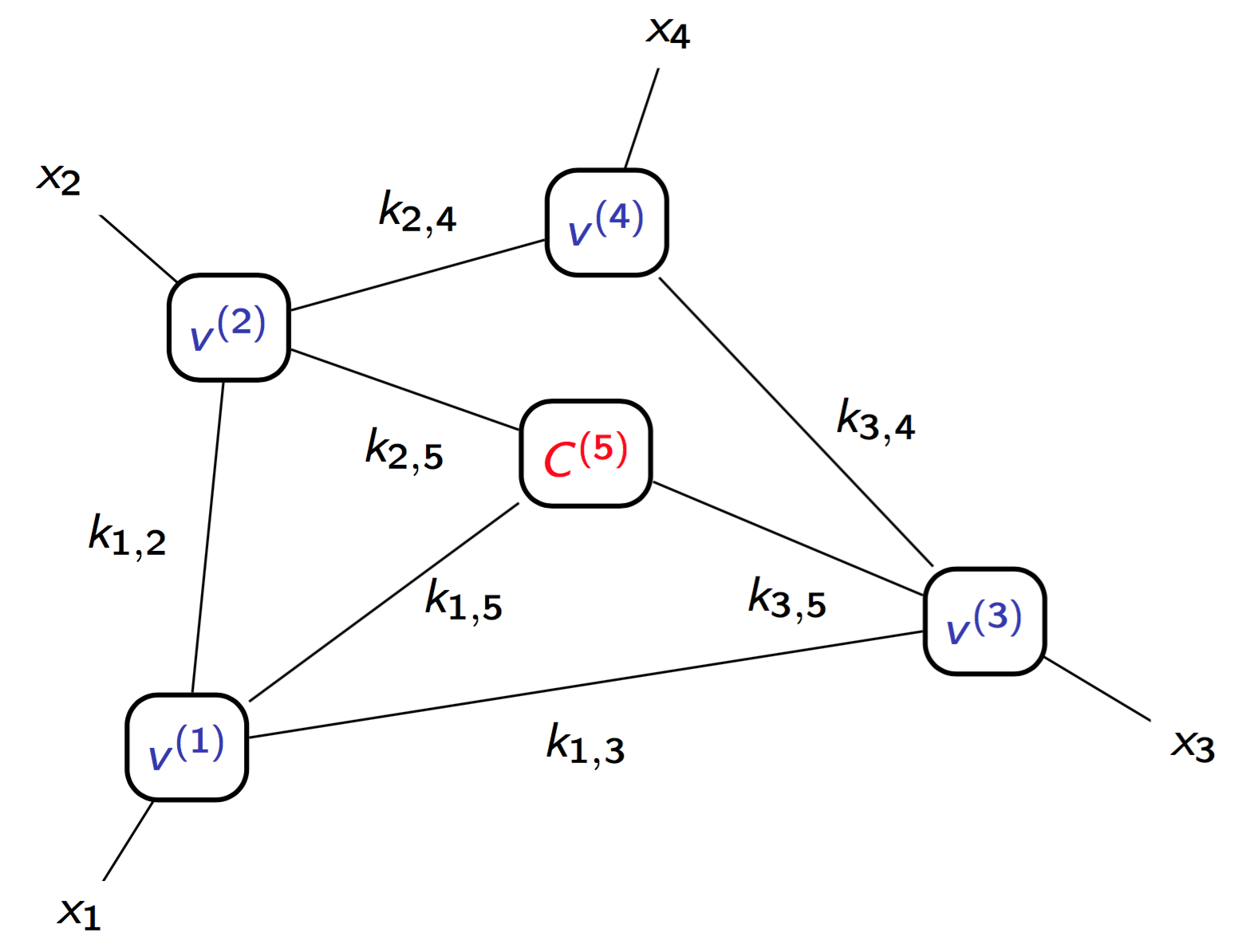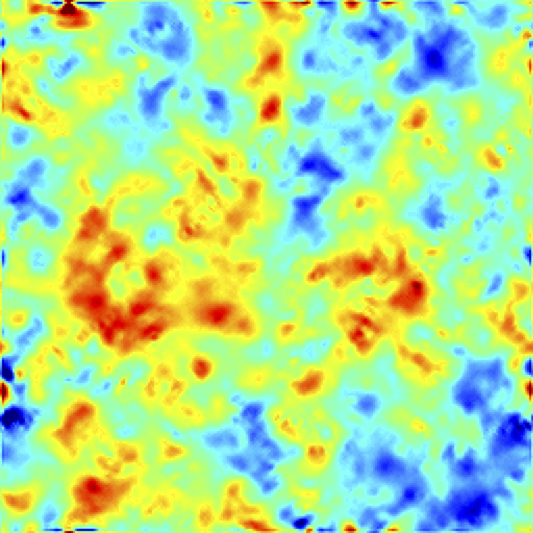# Mathematics and Applications#### Objectives

This specialisation provides a broad-based curriculum in applied mathematics, from the core notions in analysis and probability to more applied vocational concepts in statistics or scientific computing.

The multidisciplinary nature of this specialisation represents an advantage across a wide range of sectors requiring a sound understanding of mathematical tools and concepts in order to meet new technical and economic challenges. The aim is not only to provide a solid grounding in mathematics, but also a good grasp of topical issues. The lecturers lead research activities linked to different industrial sectors (aerospace, nuclear power, energy, environment, health, finance etc). This gives the students exposure to the practical applications of mathematical tools and concepts and possible career orientations.

Two tracks are on offer within the specialisation: 'Statistics and Data Science' and 'Numerical analysis and probability'.

## List of Courses

Core Courses:
• Probability
• Hilbertian analysis
• Deterministic numerical methods
• Probabilistic numerical methods
• Statistical learning
• Stochastic processes
• Uncertainty quantification
• Project
'Numerical analysis and probability' Track Courses:
• Partial differential equations
• Numerical analysis
• Stochastic modelling
• Modelling for health and biology
'Statistics and Data Science' Track Courses:
• Statistics 1
• Statistics 2
• Computational statistics
• High dimensional statistics
• Bayesian methods and hierarchical models## Examples of Past Projects

• Portfolio optimization
• Optimal uncertainty quantification for solving stochastic differential equations
• Monte Carlo methods for rare event estimation
• Statistical learning methods for metamodelling of a flight controller
• Patterns of Alan Turing
• Portfolio risk measures
• Population dynamics and breast cancer tumor growth modelling
• Data mining for the analysis of petroglyphs
• Numerical simulation of the transport of nuclear waste
• Matrix completion for painting restoration
• Multilevel Monte Carlo methods for option pricing
• Metamodelling of chaotic dynamical systems
• Study of the graph of Erdos Renyi

## Examples of past internships

• Classification and Forecasting of load curves (GDF Suez strategy division)
• Integration of external variables to optimize hotel prices (Amadeus)
• Development of a simulator (Thalès Alenia Space)
• Reporting of investment funds (Prévoir)
• Environmental characterization of the aircraft fleet (Safran)
• Actuarial problems in reinsurance (Wills Re)
• Reliability assessment of hybrid dynamical systems (EDF, Division Management of Industrial Risks)
• Reporting of market risks for gas portfolio (EDF, Division Economy, Rate and Price)
• Uncertainty quantification of Pégase (SAFRAN)
• Structure condition forecast using Markov chains (University of Nevada Las Vegas)
• Combination of statistical models for photovoltaic power forecasting (Reuniwatt)
• Optimization of a statistical tool for sale forecasting (PSA)
• Stochastic methods for the solution of high-dimensional PDEs (Ecole Centrale Nantes)
• Mathematical analysis and numerical methods for a PDE model governing a ratchet-cap pricing in the EURIBOR (Universidad Cardenal Herrera Valencia)

## Sectors of activity and employment prospects

Applied mathematics skills are in increasing demand across numerous industrial sectors, as much for the technical skills acquired through this type of course as for the abstraction and generalisation abilities developed through mathematics. Thus this specialization opens up career opportunities for students in engineering mathematics positions (data science, modelling, numerical simulation, probability, statistics and data analysis) across different sectors (aerospace, nuclear, energy, environment, health, finance).
Published on November 2, 2015 Updated on March 18, 2019